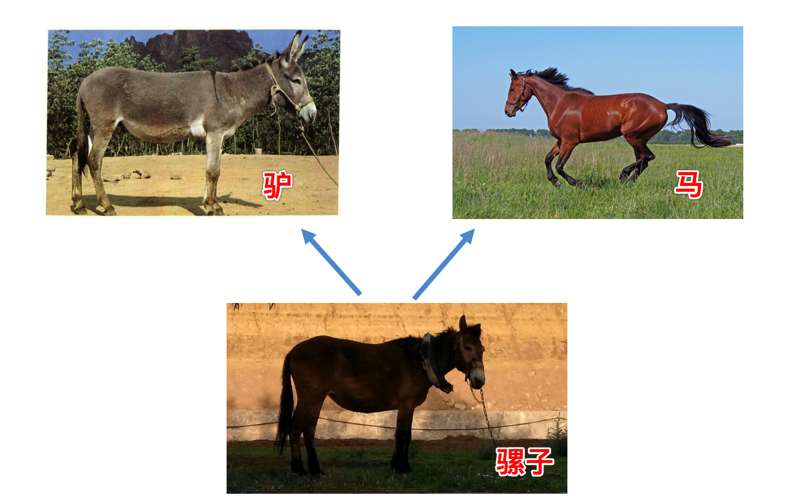o
osc_g8254g7s

# 封装

``````import time

class Clock:

def __init__(self):
# 获取当前系统时间
t = time.localtime()
self._hour = t.tm_hour
self._minute = t.tm_min
self._second = t.tm_sec

def run(self):
self._second += 1
if self._second == 60:
self._second = 0
self._minute += 1
if self._minute == 60:
self._minute = 0
self._hour += 1
if self._hour == 24:
self._hour = 0

def show(self):
return "%02d:%02d:%02d" % (self._hour, self._minute, self._second)

def main():
clock = Clock()
while True:
# 将光标退到当前行首
print("\r%s" % clock.show(), end="")
time.sleep(1)
clock.run()

if __name__ == '__main__':
main()
``````

# 继承

## 单继承

``````# 定义一个父类，如下:
class Cat(object):

def __init__(self, name, color="白色"):
self.name = name
self.color = color

def run(self):
print("%s--在跑"%self.name)

# 定义一个子类，继承Cat类如下:
class Bosi(Cat):
# 子类没有实现__init__方法，则自动调用父类的__init__方法

def setNewName(self, newName):
self.name = newName

def eat(self):
print("%s--在吃"%self.name)

bs = Bosi("印度猫")
print('bs的名字为:%s'%bs.name)
print('bs的颜色为:%s'%bs.color)
bs.eat()
bs.setNewName('波斯')
bs.run()

"""

bs的名字为:印度猫
bs的颜色为:白色

"""
``````

## 多继承``````# 定义一个父类
class A:
def printA(self):
print('----A----')

# 定义一个父类
class B:
def printB(self):
print('----B----')

# 定义一个子类，继承自A、B
class C(A,B):
def printC(self):
print('----C----')

obj_C = C()
obj_C.printA()
obj_C.printB()

"""

----A----
----B----
"""
``````

``````class A:
def print(self):
print('----A----')

class B:
def print(self):
print('----B----')

# 定义一个子类，继承自A、B
class C(A,B):
pass

obj_C = C()
obj_C.print()

"""

----A----
"""
``````

``````print(C.__mro__)
"""

(<class '__main__.C'>, <class '__main__.B'>, <class '__main__.A'>, <class 'object'>)
"""
``````

## 重写父类的方法

``````class Cat:
def sayHello(self):
print("halou-----1")

class Bosi(Cat):

def sayHello(self):
print("halou-----2")

class Bali(Cat):

def sayHello(self):
print("halou-----3")

bosi = Bosi()
bali= Bali()
bosi.sayHello()
bali.sayHello()
"""

halou-----2
halou-----3
"""
``````

## 调用父类的方法

``````class Cat:
def __init__(self,name):
self.name = name

class Bosi(Cat):

def __init__(self,name):
# 调用父类的__init__方法1(python2)
#Cat.__init__(self,name)
# 调用父类的__init__方法2
#super(Bosi,self).__init__(name)
# 调用父类的__init__方法3
super().__init__(name)
self.color = 'yellow'

def getName(self):
return self.name

bosi = Bosi('xiaohua')

print(bosi.name)
print(bosi.color)
"""

xiaohua
yellow
"""
``````

• 上文说过如果在子类中需要父类的构造方法就需要显式地调用父类的构造方法，或者不重写父类的构造方法，子类不重写 __init __，实例化子类时，会自动调用父类定义的 __init __。
• 如果子类重写了__init__ 时，实例化子类，就不会调用父类已经定义的 __init __。
• 如果重写了__init __ 时，要继承父类的构造方法，可以使用如下方法：
• super(子类，self).init(参数1，参数2，....)
• 父类名称.init(self,参数1，参数2，...)

# 多态

o

### osc_g8254g7s

Python面向对象这样理解更简单

Python虽然是解释型语言，但从设计之初就已经是一门面向对象的语言，对于Python来说一切皆为对象。正因为如此，在Python中创建一个类和对象是很容易的，当然如果习惯面向过程或者函数的写法也...

2019/01/28
0
0
Python面向对象你不知道的特点

Python虽然是解释型语言，但从设计之初就已经是一门面向对象的语言，对于Python来说一切皆为对象。正因为如此，在Python中创建一个类和对象是很容易的，当然如果习惯面向过程或者函数的写法也...

lmseo5hy
03/31
0
0
Python是面向对象的语言吗？python编程

Python虽然是解释型语言，但从设计之初就已经是一门面向对象的语言，对于Python来说一切皆为对象。正因为如此，在Python中创建一个类和对象是很容易的，当然如果习惯面向过程或者函数的写...

2018/06/08
8
0
python面向对象三大特征

osc_h51vfdqy
2019/05/04
1
0
Python 入门 之 面向对象的三大特性（封装 / 继承 / 多态）

Python 入门 之 面向对象的三大特性（封装 / 继承 / 多态） 1、面向对象的三大特性： （1）继承 继承是一种创建新类的方式，在Python中，新建的类可以继承一个或多个父类，父类又可以称为基类...

osc_lbaj0fqn
2019/09/25
3
0

MongoDB入门系列——3.可视化工具篇

2018/11/08
17
0

Exercise 10: Hierarchical clustering of the grain data In the video, you learnt that the SciPy linkage() function performs hierarchical clustering on an array of samples. Use th......

48分钟前
13
0

<input model:value="{{value}}" />

48分钟前
9
0

51分钟前
13
0
centos宝塔面板安装及常见错误处理(超级详细)

17
0